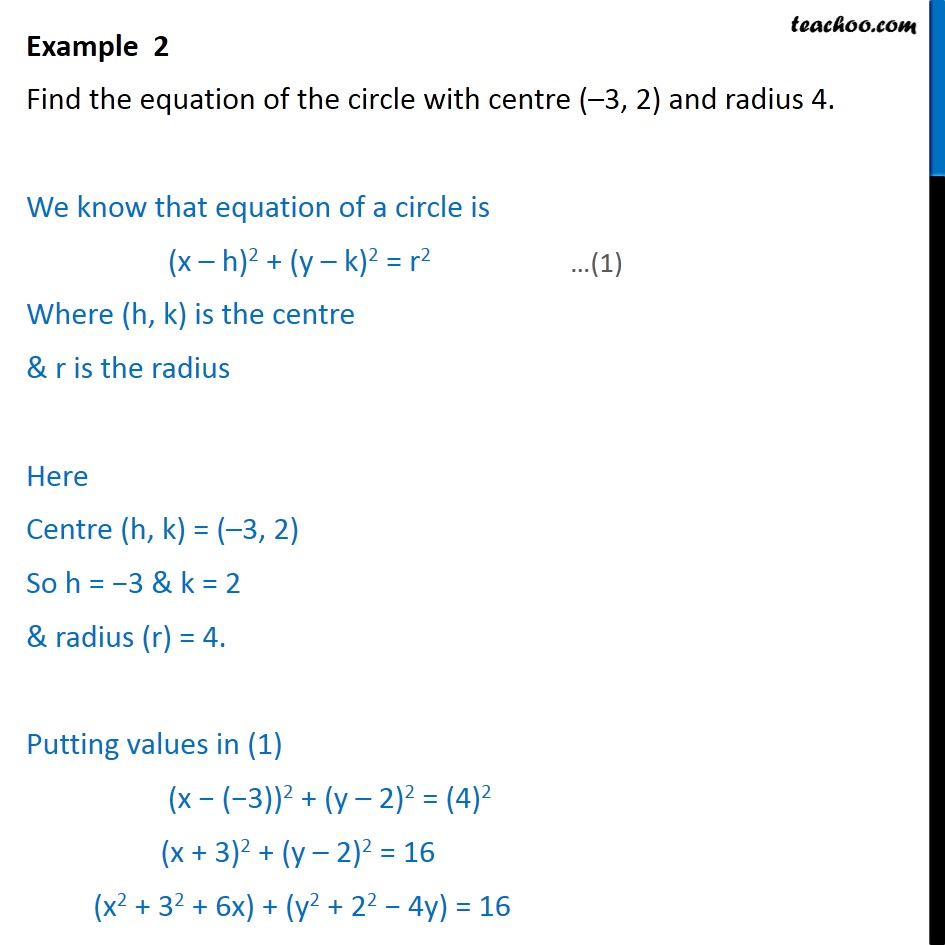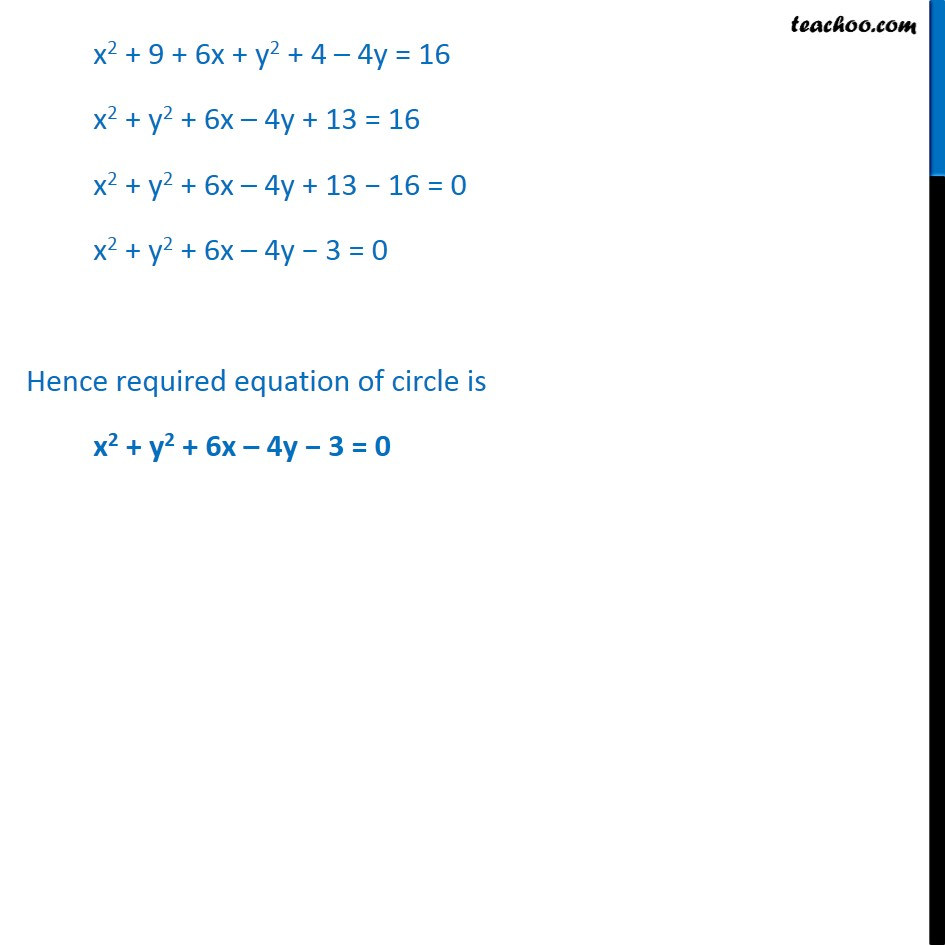Examples

Chapter 11 Class 11 Conic Sections (Term 2)
Serial order wise### Transcript

Example 2 Find the equation of the circle with centre (–3, 2) and radius 4. We know that equation of a circle is (x­ – h)2 + (y ­– k)2 = r2 Where (h, k) is the centre & r is the radius Here Centre (h, k) = (–3, 2) So h = −3 & k = 2 & radius (r) = 4. Putting values in (1) (x − (−3))2 + (y – 2)2 = (4)2 (x + 3)2 + (y – 2)2 = 16 (x2 + 32 + 6x) + (y2 + 22 − 4y) = 16 x2 + 9 + 6x + y2 + 4 – 4y = 16 x2 + y2 + 6x – 4y + 13 = 16 x2 + y2 + 6x – 4y + 13 − 16 = 0 x2 + y2 + 6x – 4y − 3 = 0 Hence required equation of circle is x2 + y2 + 6x – 4y − 3 = 0